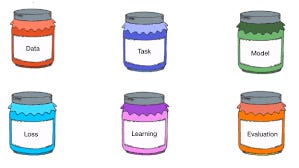# Elements of Machine Learning

Source: Deep Learning on Medium

Machine Learning,here on referred as ML can be understood in different ways.One of the way is 6 jars that was taught to me in one fourth labs.ML process can be divided into 6 elements ,which are referred to as jars in this article.Let’s dive into these 6 jars.

1. DATA:Collecting Data is the first and foremost thing.Data can be of any type, like image,video,speech,text etc.This raw data must be converted into to numerical values so that a machine can understand the data.For supervised learning,the data must collect the inputs and outputs of previous cases,using which machine learns and predict solution for any new data.For unsupervised learning,only the inputs are sufficient.So,our first jar will be DATA.
2. TASKS:After collecting the data,the next thing is to define the tasks.In the given data,one can relate any of the attributes. Defining the x(input) and y(output) will be the task.This will be our second jar.
3. MODELS:After deciding the task, many models can be proposed. Predicting the model is nothing but finding f(x) in the relation y=f(x)*x. f(x) can be any function, like trigonometric,logarithmic,exponential etc.This will be our 3rd jar.
4. LOSS FUNCTION:Loss function is the measure of how bad a model is,higher the value of loss,bad is the model.Loss can be evaluated using many functions.Some of them are Squared error loss,Cross Entropy loss,KL Divergence loss etc.Loss is our 4th jar
5. LEARNING ALGORITHM:Learning Algorithms are used to evaluate the parameters in the models.Learning Algorithms like Gradient Descent,Adagrad,RMSProp,Adam etc are used to compute the parameters in model. Learning Algorithms are used to minimize the loss function.
6. EVALUATION:One can think that Loss function is enough to say whether a model is good or bad which is not correct.Evaluation of model must be done to say whether a model is good or bad.Here evaluation is knowing the accuracy of the model.The percentage of it’s success is the accuracy of a model.Sometimes,a model which has minimum loss cannot not predict correctly,which is not what we want.So,accuracy is used to evaluate a ML model.This concludes our 6th jar.6 JARS OF MACHINE LEARNING